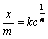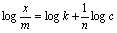1800-1023-196

+91-120-4616500

(1) The process of adsorption can take place from solutions also.

(2) In any solution, there are two (or more) components ; solute and solvent. The solute may be present in the molecular or ionic form.

(3) The extent of adsorption from solution depends upon the concentration of the solute in the solution, and can be expressed by the Freundlich isotherm.

(4) The Freundlich adsorption isotherm for the adsorption from solution is,where, x is the mass of the solute adsorbed, m is the mass of the solid adsorbent, c is the equilibrium concentration of the solute in the solution, n is a constant having value greater than one,

k is the proportionality constant, (The value of k depends upon the nature of solid, its particle size, temperature, and the nature of solute and solvent etc.)

(5) The plot of x/m against c is similar to that Freundlich adsorption isotherm. The above equations may be written in the following form,where c, is the equilibrium concentration of the solute in the solution.

## NEET & AIIMS Exam Sample Papers

 AIIMS SAMPLE PAPERS View More NEET SAMPLE PAPERS View More